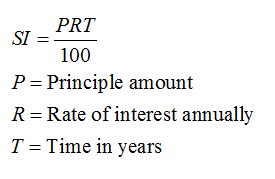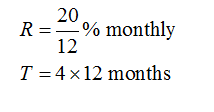# Ken wants a \$1000 TV/sound system. He can't afford that amount so he uses his credit card with 20% annual interest. If he only pays interest each month and that credit card debt about how much will he pay in interest in 4 years?

Question
283 views

Ken wants a \$1000 TV/sound system. He can't afford that amount so he uses his credit card with 20% annual interest. If he only pays interest each month and that credit card debt about how much will he pay in interest in 4 years?

check_circle

Step 1

First of all, consider the SI formula for the calculation of the interest monthly.

Principal amount = \$ 1000

Rate of interest = 20%

Time = 4 yearsStep 2

Now, calculate the rate of interest and time for the monthly payment of the interest.

Divide the rate of interest by 12 to calculate the rate of interest monthly.

Multiply the time by 12 to calculate the time in months....

### Want to see the full answer?

See Solution

#### Want to see this answer and more?

Solutions are written by subject experts who are available 24/7. Questions are typically answered within 1 hour.*

See Solution
*Response times may vary by subject and question.
Tagged in

### Algebra